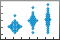# swarmchart

•## 语法

``swarmchart(x,y)``
``swarmchart(x,y,sz)``
``swarmchart(x,y,sz,c)``
``swarmchart(___,mkr)``
``swarmchart(___,'filled')``
``swarmchart(tbl,xvar,yvar)``
``swarmchart(tbl,xvar,yvar,'filled')``
``swarmchart(ax,___)``
``swarmchart(___,Name,Value)``
``s = swarmchart(___)``

## 说明

### 向量和矩阵数据

````swarmchart(x,y)` 显示一个分簇散点图，它是一个散点图，点在 `x` 维度中偏移（抖动）。这些点形成不同形状，每个形状的轮廓类似于小提琴图。分簇散点图有助于您可视化离散的 `x` 数据以及 `y` 数据的分布。在 `x` 中的每个位置，点根据 `y` 的核密度估计值发生抖动。 要绘制一组点，请将 `x` 和 `y` 指定为等长向量。要在同一组坐标区上绘制多组点，请将 `x` 或 `y` 中的至少一个指定为矩阵。 ```

``swarmchart(x,y,sz)` 指定标记大小。要以相同的大小绘制所有标记，请将 `sz` 指定为标量。要绘制具有不同大小的标记，请将 `sz` 指定为向量或矩阵。`

``swarmchart(x,y,sz,c)` 指定标记颜色。您可以为所有标记指定一种颜色，也可以更改颜色。例如，您可以通过将 `c` 指定为 `'red'` 来绘制所有红色圆。`

``swarmchart(___,mkr)` 指定不同于默认标记（圆形）的标记。需要在上述任一语法中的所有参数之后指定 `mkr`。`

``swarmchart(___,'filled')` 填充标记。需要在上述任一语法中的所有参数之后指定 `'filled'` 选项。`

### 表数据

``swarmchart(tbl,xvar,yvar)` 绘制表 `tbl` 中的变量 `xvar` 和 `yvar`。要绘制一个数据集，请为 `xvar` 指定一个变量，为 `yvar` 指定一个变量。要绘制多个数据集，请为 `xvar`、`yvar` 或两者指定多个变量。如果两个参数都指定多个变量，它们指定的变量数目必须相同。`

``swarmchart(tbl,xvar,yvar,'filled')` 绘制指定的变量并填充标记。`

### 其他选项

``swarmchart(ax,___)` 在目标坐标区中显示分簇散点图。需要在上述任一语法中的所有参数之前指定坐标区。`

````swarmchart(___,Name,Value)` 使用一个或多个名称-值参数指定群图的其他属性。在所有其他输入参数后指定属性。例如： `swarmchart(x,y,'LineWidth',2)` 创建一个具有两点标记轮廓的分簇散点图。`swarmchart(tbl,'MyX','MyY','ColorVariable','MyColors')` 根据表中的数据创建一个分簇散点图，并使用表中的数据自定义标记颜色。 有关属性列表，请参阅 Scatter 属性。```

``s = swarmchart(___)` 返回 `Scatter` 对象或 `Scatter` 对象数组。创建图后，使用 `s` 修改图属性。有关属性列表，请参阅 Scatter 属性。`

## 示例

```x = [ones(1,500) 2*ones(1,500) 3*ones(1,500)]; y1 = 2 * randn(1,500); y2 = 3 * randn(1,500) + 5; y3 = 5 * randn(1,500) + 5; y = [y1 y2 y3]; swarmchart(x,y)``````x1 = ones(1,500); x2 = 2 * ones(1,500); x3 = 3 * ones(1,500); y1 = 2 * randn(1,500); y2 = [randn(1,250) randn(1,250) + 4]; y3 = 5 * randn(1,500) + 5;```

```swarmchart(x1,y1,5) hold on swarmchart(x2,y2,5) swarmchart(x3,y3,5) hold off````BicycleCounts.csv` 数据集读入名为 `tbl` 的时间表中。此数据集包含一段时间内的自行车交通流量数据。显示 `tbl` 的前五行。

```tbl = readtable("BicycleCounts.csv"); tbl(1:5,:)```
```ans=5×5 table Timestamp Day Total Westbound Eastbound ___________________ _____________ _____ _________ _________ 2015-06-24 00:00:00 {'Wednesday'} 13 9 4 2015-06-24 01:00:00 {'Wednesday'} 3 3 0 2015-06-24 02:00:00 {'Wednesday'} 1 1 0 2015-06-24 03:00:00 {'Wednesday'} 1 1 0 2015-06-24 04:00:00 {'Wednesday'} 1 1 0 ```

```daynames = ["Sunday" "Monday" "Tuesday" "Wednesday" "Thursday" "Friday" "Saturday"]; x = categorical(tbl.Day,daynames); y = tbl.Total; swarmchart(x,y,'.');````BicycleCounts.csv` 数据集读入名为 `tbl` 的时间表中。创建一个向量 `x`，其中包含每个观测值的星期几信息；创建另一个向量 `y`，其中包含观测到的自行车交通流量；创建第三个向量 `c`，其中包含一天中的小时信息。

```tbl = readtable("BicycleCounts.csv"); daynames = ["Sunday" "Monday" "Tuesday" "Wednesday" "Thursday" "Friday" "Saturday"]; x = categorical(tbl.Day,daynames); y = tbl.Total; c = hour(tbl.Timestamp); swarmchart(x,y,20,c,'filled');````BicycleCounts.csv` 数据集读入名为 `tbl` 的时间表中。创建一个向量 `x`，其中包含每个观测值的星期几信息；创建另一个向量 `y`，其中包含观测到的自行车交通流量；创建第三个向量 `c`，其中包含一天中的小时信息。然后创建一个关于 `x``y` 的分簇散点图，指定标记大小为 `5`，标记的颜色为向量 `c`。调用带返回参数 `s``swarmchart` 函数，以便在创建图后修改它。

```tbl = readtable("BicycleCounts.csv"); daynames = ["Sunday" "Monday" "Tuesday" "Wednesday" "Thursday" "Friday" "Saturday"]; x = categorical(tbl.Day,daynames); y = tbl.Total; c = hour(tbl.Timestamp); s = swarmchart(x,y,5,c);``````s.XJitter = 'rand'; s.XJitterWidth = 0.5;``````x1 = ones(1,500); x2 = 2 * ones(1,500); x = [x1 x2]; y1 = 2 * randn(1,500); y2 = [randn(1,250) randn(1,250) + 4]; y = [y1 y2]; swarmchart(x,y,'filled','MarkerFaceAlpha',0.5,'MarkerEdgeAlpha',0.5)``````tbl = table(randi(2,100,1),randn(100,1),randn(100,1)+10, ... 'VariableNames',{'X','Y1','Y2'}); swarmchart(tbl,'X','Y1')``````swarmchart(tbl,'X',{'Y1','Y2'}) legend``````tbl = table(randi(2,100,1),randn(100,1),randn(100,1), ... 'VariableNames',{'X','Y','Colors'}); s = swarmchart(tbl,'X','Y','filled','ColorVariable','Colors');````s.SizeData = 100;``BicycleCounts.csv` 数据集读入名为 `tbl` 的时间表中。此数据集包含一段时间内的自行车交通流量数据。显示 `tbl` 的前五行。

```tbl = readtable("BicycleCounts.csv"); tbl(1:5,:)```
```ans=5×5 table Timestamp Day Total Westbound Eastbound ___________________ _____________ _____ _________ _________ 2015-06-24 00:00:00 {'Wednesday'} 13 9 4 2015-06-24 01:00:00 {'Wednesday'} 3 3 0 2015-06-24 02:00:00 {'Wednesday'} 1 1 0 2015-06-24 03:00:00 {'Wednesday'} 1 1 0 2015-06-24 04:00:00 {'Wednesday'} 1 1 0 ```

`x` 定义为表中表示周中日期的分类数组。将 `yEast``yWest` 定义为包含东行和西行自行车交通流量计数的向量。

```daynames = ["Sunday" "Monday" "Tuesday" "Wednesday" "Thursday" "Friday" "Saturday"]; x = categorical(tbl.Day,daynames); yEast = tbl.Eastbound; yWest = tbl.Westbound;```

```tiledlayout('flow') ax1 = nexttile; y = tbl.Eastbound; swarmchart(ax1,x,y,'.')``````ax2 = nexttile; y = tbl.Westbound; swarmchart(ax2,x,y,'.')```## 输入参数

x 坐标，指定为标量、向量或矩阵。`x` 的大小和形状取决于数据的形状。下表说明了最常见的情况。

`x``y` 指定为标量。例如：

`swarmchart(1,1)`

```x = randi(3,100,1); y = randn(1,100); swarmchart(x,y)```

```x = randi(2,1,100); y = [randn(100,1) randn(100,1)+5]; swarmchart(x,y,100)```

```x = randi(2,100,2); y = [randn(100,1) randn(100,1)+5]; swarmchart(x,y,100)```

y 坐标，指定为标量、向量或矩阵。`y` 的大小和形状取决于数据的形状。下表说明了最常见的情况。

`x``y` 指定为标量。例如：

`swarmchart(1,1)`

```x = randi(3,100,1); y = randn(1,100); swarmchart(x,y)```

```x = randi(2,1,100); y = [randn(100,1) randn(100,1)+5]; swarmchart(x,y,100)```

```x = randi(2,100,2); y = [randn(100,1) randn(100,1)+5]; swarmchart(x,y,100)```

`x` 指定为向量，将 `y` 指定为矩阵，将 `sz` 指定为标量。

```x = randi(2,1,100); y = randn(100,1); swarmchart(x,y,100)```

• `x``y` 长度相同的向量。

• 有至少一个维度与 `x``y` 长度匹配的矩阵。指定矩阵对于在每个 (x,y) 位置显示具有不同大小的多个标记非常有用。

`x``y``sz` 指定为向量。

```x = randi(2,1,100); y = randn(100,1); sz = randi([70 2000],100,1); swarmchart(x,y,sz)```

`x``y` 指定为向量，将 `sz` 指定为矩阵。

```x = randi(2,1,100); y = randn(100,1); sz = randi([70 2000],100,2); swarmchart(x,y,sz)```

`x``y` 中有至少一个是用于绘制多个数据集的矩阵

• 其元素数与每个数据集中的点数相同的向量。

• `x``y` 矩阵大小相同的矩阵。

`x` 指定为向量，将 `y` 指定为矩阵，将 `sz` 指定为向量。

```x = randi(2,1,100); y = [randn(100,1) randn(100,1)+5]; sz = randi([70 2000],100,1); swarmchart(x,y,sz)```

`x` 指定为向量，将 `y` 指定为矩阵，将 `sz` 指定为与 `y` 大小相同的矩阵。

```x = randi(2,1,100); y = [randn(100,1) randn(100,1)+5]; sz = randi([70 2000],100,2); swarmchart(x,y,sz)```

• 颜色名称 - 颜色名称（如 `'red'`）或短名称（如 `'r'`）。

• RGB 三元组 - 三元素行向量，其元素分别指定颜色中红、绿、蓝分量的强度。强度值必须位于 `[0,1]` 范围内，例如 `[0.4 0.6 0.7]`。RGB 三元组对于创建自定义颜色很有用。

• RGB 三元组矩阵 - 三列矩阵，其中的每一行均为一个 RGB 三元组。

• 由颜色图索引组成的向量 - 数值向量，其长度与 `x``y` 向量相同。

```x = randi(2,1,100); y = randn(100,1); c = 'red'; swarmchart(x,y,[],c)```

```x = randi(2,1,100); y = randn(100,1); c = [0.6 0 0.9]; swarmchart(x,y,[],c)```

```x = randi(2,1,100); y = randn(100,1); c = 1:100; swarmchart(x,y,[],c) colormap(gca,'winter')```

```x = randi(2,1,100); y = randn(100,1); c = rand(100,3); swarmchart(x,y,[],c)```

```x = randi(2,100,2); y = [randn(100,1) randn(100,1)+5]; c = [1 0 0; 0 0 1]; swarmchart(x,y,[],c)```

#### 常见颜色的颜色名称和 RGB 三元组

`"red"``"r"``[1 0 0]``"#FF0000"``"green"``"g"``[0 1 0]``"#00FF00"``"blue"``"b"``[0 0 1]``"#0000FF"``"cyan"` `"c"``[0 1 1]``"#00FFFF"``"magenta"``"m"``[1 0 1]``"#FF00FF"``"yellow"``"y"``[1 1 0]``"#FFFF00"``"black"``"k"``[0 0 0]``"#000000"``"white"``"w"``[1 1 1]``"#FFFFFF"`RGB 三元组十六进制颜色代码外观
`[0 0.4470 0.7410]``"#0072BD"``[0.8500 0.3250 0.0980]``"#D95319"``[0.9290 0.6940 0.1250]``"#EDB120"``[0.4940 0.1840 0.5560]``"#7E2F8E"``[0.4660 0.6740 0.1880]``"#77AC30"``[0.3010 0.7450 0.9330]``"#4DBEEE"``[0.6350 0.0780 0.1840]``"#A2142F"``"o"`圆圈`"+"`加号`"*"`星号`"."``"x"`叉号`"_"`水平线条`"|"`垂直线条`"square"`方形`"diamond"`菱形`"^"`上三角`"v"`下三角`">"`右三角`"<"`左三角`"pentagram"`五角形`"hexagram"`六角形`'filled'` 选项将 `Scatter` 对象的 `MarkerFaceColor` 属性设置为 `'flat'`，并将 `MarkerEdgeColor` 属性设置为 `'none'`。在这种情况下，MATLAB 绘制标记面，但不绘制边。

#### 指定表索引

• 字符串、字符向量或元胞数组。

• `"A"``'A'` - 名为 `A` 的变量

• `["A","B"]``{'A','B'}` - 名为 `A``B` 的两个变量

• `"Var"+digitsPattern(1)` - 变量名为 `"Var"` 后跟一个数字

• 引用变量在表中位置的索引编号。

• 由数字组成的向量。

• 逻辑向量。通常，此向量的长度与变量的数目相同，但可以省略尾部的 `0``false` 值。

• `3` - 表中的第三个变量

• `[2 3]` - 表中的第二个和第三个变量

• `[false false true]` - 第三个变量

• `vartype("categorical")` - 包含分类值的所有变量

#### 绘制您的数据

```tbl = table(randn(100,1),randn(100,1)+10,randn(100,1), ... 'VariableNames',{'X1','X2','Y'}); swarmchart(tbl,'X1','Y')```

`swarmchart(tbl,{'X1','X2'},'Y')`

`swarmchart(tbl,'X1',3)`

#### 指定表索引

• 字符串、字符向量或元胞数组。

• `"A"``'A'` - 名为 `A` 的变量

• `["A","B"]``{'A','B'}` - 名为 `A``B` 的两个变量

• `"Var"+digitsPattern(1)` - 变量名为 `"Var"` 后跟一个数字

• 引用变量在表中位置的索引编号。

• 由数字组成的向量。

• 逻辑向量。通常，此向量的长度与变量的数目相同，但可以省略尾部的 `0``false` 值。

• `3` - 表中的第三个变量

• `[2 3]` - 表中的第二个和第三个变量

• `[false false true]` - 第三个变量

• `vartype("categorical")` - 包含分类值的所有变量

#### 绘制您的数据

```tbl = table(randn(100,1),randn(100,1)+10,randn(100,1), ... 'VariableNames',{'X1','X2','Y'}); swarmchart(tbl,'X1','Y')```

`swarmchart(tbl,{'X1','X2'},'Y')`

`swarmchart(tbl,'X1',3)`

### 名称-值参数

• `'none'` - 不抖动点。

• `'density'` - 使用二维图的 y 的内核密度估计值对点进行抖动。如果在三维图的两个维度中指定此选项，则点会根据第三个维度中的内核密度估计值抖动。例如，将 `XJitter``YJitter` 设置为 `'density'` 会使用 z 的内核密度估计值。

• `'rand'` - 以均匀分布随机抖动点。

• `'randn'` - 以正态分布随机抖动点。

`XJitterWidth = 0.9 * min(diff(unique(x)));`

#### 指定表索引

• `"A"``'A'` - 名为 `A` 的变量

• `"Var"+digitsPattern(1)` - 名称为 `"Var"` 的变量后跟一位数字

• 引用变量在表中位置的索引编号。

• 逻辑向量。通常，此向量的长度与变量的数目相同，但可以省略尾部的 `0``false` 值。

• `3` - 表中的第三个变量

• `[false false true]` - 第三个变量

• `vartype` 下标，用于选择指定类型的表变量。该下标只能引用一个变量。

• `vartype("double")` - 包含双精度值的变量

#### 指定颜色数据

• 线性映射到当前颜色图中的一列数字。

• 由 RGB 三元组组成的一个三列数组。RGB 三元组是包含三个元素的向量，其元素值指定特定颜色的红、绿和蓝分量的强度。强度必须处于范围 `[0,1]` 中。例如，`[0.5 0.7 1]` 指定浅蓝色。

## 算法

`spread = 0.9 * min(diff(unique(x)));`